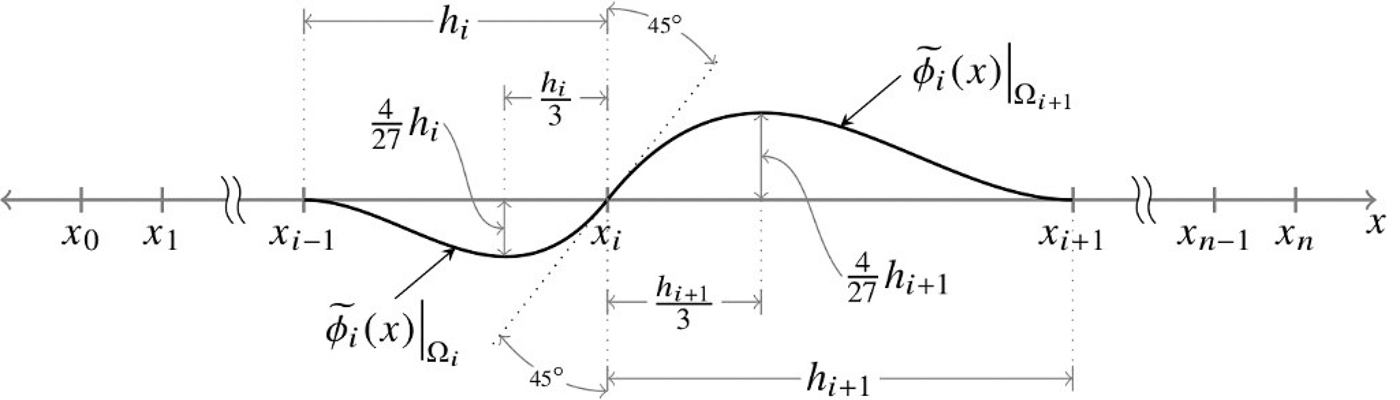# Numerical analysis and scientific computing

Our researchers develop and analyse algorithms that compute numerical approximations and apply them to real-world problems.

## PhD projects

We welcome applications for PhD study in all areas of numerical analysis and scientific computing.

PhD enquiries related to this theme can be directed to Dr Stefan Güttel.

We design algorithms for the solution of continuous problems, which requires the development of numerical approximations when closed form solutions do not exist.  These continuous problems can arise from mathematical models of physical processes such as fluid flow, heat transfer and solid mechanics.  We also treat finite-dimensional matrix problems, which typically arise from discretization of continuous operators or from data-driven applications.

Our work covers the whole spectrum of the discipline from fundamental theory to algorithms to implementation in open source software.

Our research focuses particularly on

• Approximation theory
• Fast solvers for partial differential equations
• Finite element approximation
• Numerical algorithms and software development
• Numerical linear algebra
• Uncertainty quantification for partial differential equations

We have close links with international companies such as Arup, NAG, and The MathWorks.

## Research seminars

Research seminars on topics associated with numerical analysis and scientific computing take place regularly in the following series: